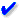## /* Neon Lights Text By JavaScript Kit (http://javascriptkit.com) For this script, TOS, and 100s more DHTML scripts, Visit http://www.dynamicdrive.com */ var message="!TECHNICALSYMPOSIUM.COM!" var neonbasecolor="ORANGE" var neontextcolor="NAVY" var flashspeed=100 //in milliseconds ///No need to edit below this line///// var n=0 if (document.all||document.getElementById){ document.write('<font color="'+neonbasecolor+'">') for (m=0;m<message.length;m++) document.write('<span id="neonlight'+m+'">'+message.charAt(m)+'</span>') document.write('</font>') } else document.write(message) function crossref(number){ var crossobj=document.all? eval("document.all.neonlight"+number) : document.getElementById("neonlight"+number) return crossobj } function neon(){ //Change all letters to base color if (n==0){ for (m=0;m<message.length;m++) //eval("document.all.neonlight"+m).style.color=neonbasecolor crossref(m).style.color=neonbasecolor } //cycle through and change individual letters to neon color crossref(n).style.color=neontextcolor if (n<message.length-1) n++ else{ n=0 clearInterval(flashing) setTimeout("beginneon()",1500) return } } function beginneon(){ if (document.all||document.getElementById) flashing=setInterval("neon()",flashspeed) } beginneon()What's New-Given Below# Entrance Exam Question Papers/Syllabus# NIMCET Syllabus

Mathematics: (40 Questions)

Set Theory:

Concepts of sets – Union – Intersection – Cardinality – Elementary counting permutations and combinations

Probability and Statistics:

Basic concepts of probability theory, Averages, Dependent and independent events, frequency distributions, measures of central tendencies and dispersions.

Algebra:

Fundamental operations in Algebra, Expansions, Factorization, simultaneous linear / quadratic equations, indices, logarithms, arithmetic, geometric and harmonic progressions, determinants and matrices.

Coordinate Geometry:

Rectangular Cartesian coordinates, distance formulae, equation of a line, intersection of lines, pair of straight lines, equations of a circle, parabola, ellipse, and hyperbola.

Calculus:

Limit of functions, Continuous function, Differentiation of function, tangents and normals, simple examples of maxima and minima. Integration of function by parts, by substitution and by partial fraction; Definite integrals, Applications of Definite Integrals to areas.

Vectors:

Position vector, addition and subtraction of vectors, scalar and vector products and their applications to simple geometrical problems and mechanics.

Trigonometry:

Simple identities, trigonometric equations, properties of triangles, solution of triangles, heights and distances, General solutions of trigonometric equations.

Analytical Ability and Logical Reasoning: (50 Questions)

The questions in this section will cover logical reasoning and quantitative aptitude. Some of the questions will be on comprehension of a logical situation and questions based on the facts given in the passage.

Computer Awareness: (15 questions)

Computer Basics: Organization of a Computer, Central Processing Unit (CPU), Structure of instructions in CPU, input/output devices, computer memory, back-up devices.

Data Representation:

Representation of characters, integers and fractions, binary and hexadecimal representations, Binary Arithmetic: Addition, subtraction, multiplication, division, simple arithmetic and two’s complement arithmetic, floating point representation of numbers, Boolean algebra, truth tables, Venn diagrams. General Engilish: (15 questions)

Questions in this section will be designed to test the candidates’ general understanding of the English language. There will be questions on the following topics. Comprehension, Vocabulary, Basic English Grammar (like usage of correct forms of verbs, prepositions and articles). Word power, Synonyms and Antonyms, Meanings of words and phrases, Technical writing.

# Technicalsymposium.com- All Information Contents Given Below-Click & Get All Details